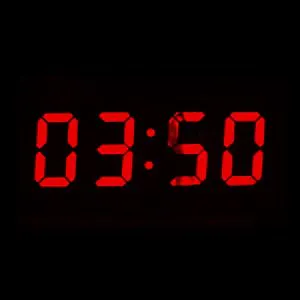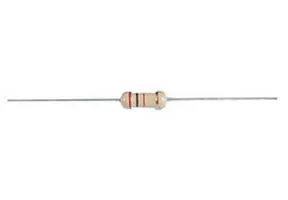Project tutorialArduino Clock © CC BY-NC-SA

This lesson aims to show how to make a simple Arduino based clock using an a LCD and a real time clock.

• 28,398 views
• 14 respects

Components and suppliesArduino UNO & Genuino UNO
×1
 PCF8563
×1
 Crystal 32kHz
×1
 10K potentiometers
×10
 10k 1/4w resistor
×10Resistor 220 ohm
×10
 LCD 16x2
×1
 Breadboard 830 points with Wire Kit
×1

1. Before you start

In this lesson will be used an LCD and real time clock, so take care to read the lessons Arduino & LCDs and Real Time Clock (RTC) PCF8563 before you start.

2. Schematic

The schematic is a merge of the two previous lessons so it is very simple. The PCF8563 communicate with Arduino using TWI and when the data is received the LCD is refreshed, showing the new time.

3. Set date and time

To set Date and Time use the following sketch:

/* Demonstration of Rtc_Pcf8563 Set Time.
* Set the clock to a time then loop over reading time and
* output the time and date to the serial console.
*
* I used a RBBB with Arduino IDE, the pins are mapped a
* bit differently. Change for your hw
* SCK - A5, SDA - A4, INT - D3/INT1
*
* After loading and starting the sketch, use the serial monitor
* to see the clock output.
*
* setup:  see Pcf8563 data sheet.
*         1x 10Kohm pullup on Pin3 INT
*         No pullups on Pin5 or Pin6 (I2C internals used)
*         1x 0.1pf on power
*         1x 32khz chrystal
*
* Joe Robertson, jmr
* orbitalair@bellsouth.net
*/

#include <Wire.h>
#include <Rtc_Pcf8563.h>

//init the real time clock
Rtc_Pcf8563 rtc;

void setup()
{
//clear out the registers
rtc.initClock();
//day, weekday, month, century(1=1900, 0=2000), year(0-99)
rtc.setDate(14, 6, 3, 1, 10);
//hr, min, sec
rtc.setTime(1, 15, 0);
}

void loop()
{
//both format functions call the internal getTime() so that the
//formatted strings are at the current time/date.
Serial.print(rtc.formatTime());
Serial.print("\r\n");
Serial.print(rtc.formatDate());
Serial.print("\r\n");
delay(1000);
}

4. The code

#include <Wire.h>
#include <Rtc_Pcf8563.h>

//init the real time clock
Rtc_Pcf8563 rtc;

// include the library code:
#include

// initialize the library with the numbers of the interface pins
LiquidCrystal lcd(12, 11, 5, 4, 3, 2);

void setup() {
// set up the LCD's number of columns and rows:
lcd.begin(16, 2);
lcd.print(" Arduino Clock!");
while(1500 - millis() > 0);
pinMode(13, OUTPUT);
}

void loop() {
lcd.setCursor(0, 0);
lcd.print("Date: ");
lcd.print(rtc.formatDate());

lcd.setCursor(0, 1);
lcd.print("Time: ");
lcd.print(rtc.formatTime());
}

Code

Code snippet #1Arduino
/* Demonstration of Rtc_Pcf8563 Set Time.
* Set the clock to a time then loop over reading time and
* output the time and date to the serial console.
*
* I used a RBBB with Arduino IDE, the pins are mapped a
* bit differently. Change for your hw
* SCK - A5, SDA - A4, INT - D3/INT1
*
* After loading and starting the sketch, use the serial monitor
* to see the clock output.
*
* setup:  see Pcf8563 data sheet.
*         1x 10Kohm pullup on Pin3 INT
*         No pullups on Pin5 or Pin6 (I2C internals used)
*         1x 0.1pf on power
*         1x 32khz chrystal
*
* Joe Robertson, jmr
* orbitalair@bellsouth.net
*/
#include <Wire.h>
#include <Rtc_Pcf8563.h>

//init the real time clock
Rtc_Pcf8563 rtc;

void setup()
{
//clear out the registers
rtc.initClock();
//day, weekday, month, century(1=1900, 0=2000), year(0-99)
rtc.setDate(14, 6, 3, 1, 10);
//hr, min, sec
rtc.setTime(1, 15, 0);
}

void loop()
{
//both format functions call the internal getTime() so that the
//formatted strings are at the current time/date.
Serial.print(rtc.formatTime());
Serial.print("\r\n");
Serial.print(rtc.formatDate());
Serial.print("\r\n");
delay(1000);
}
Code snippet #2Arduino
#include <Wire.h>
#include <Rtc_Pcf8563.h>
// include the RTC library
#include <LiquidCrystal.h>

//init the real time clock
Rtc_Pcf8563 rtc;

// initialize the library with the numbers of the interface pins
LiquidCrystal lcd(12, 11, 5, 4, 3, 2);

void setup() {
// set up the LCD's number of columns and rows:
lcd.begin(16, 2);
lcd.print(" Arduino Clock!");
while(1500 - millis() > 0);
pinMode(13, OUTPUT);
}

void loop() {
lcd.setCursor(0, 0);
lcd.print("Date: ");
lcd.print(rtc.formatDate());

lcd.setCursor(0, 1);
lcd.print("Time: ");
lcd.print(rtc.formatTime());
}

SchematicsAdjusting Dual Clock using DS3231 on 1.8" ST7735 Display

Project showcase by FLORICA Tudor-Nicusor

• 3,966 views
• 11 respects

MultiFunctional Clock

Project in progress by 3 developers

• 15,853 views
• 28 respects

Complete Digital Clock Including Alarm and Motion Sensor

Project tutorial by LAGSILVA

• 12,678 views
• 50 respects

eDOT - Arduino Based Precision Clock and Weather Station

Project tutorial by antiElectron

• 9,119 views
• 24 respects

Analog Clock using 1Sheeld Graphical LCD

Project tutorial by Ahmed El-Hinidy

• 2,069 views